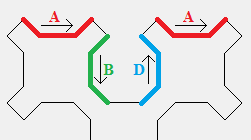Yep, Sierpinski's curve, not the more famous triangle. It's a form of space-filling curve and is quite enjoyable to look at.

## Existing solutions

So, this isn’t a rarely seen fractal, but a particular variant of it is. There are many ways to implement this fractal. Many of those implementations that I found online were of this variety, where you had a distinct drawing function for each 4 types of edges.Implementations I found relied on hardcoding each type of curve source

I love seeing different approaches to the same problem and much respect to all the people who published their code from which I learned a lot. However, I was convinced there had to be a more elegant way. It came in the form of a Youtube video from Eddie Woo.

It’s such an elegant explanation for space filling curces and in particular the Sierpinski’s curve, by thinking about it as a subdivision of space. I highly recommend all 4 parts of the space-filling curve series.

The advantage of this implementation over the existing implementations is that it also surfaces the intermediary iterations, the ones that have edges that look like bulbs, in addition to the classic Sierpinski’s curve shape.left: 6th iteration with the 'bulbs'. right: 7th iteration, classic Sierpinski's curve shape, where 'bulbs' appear in negative space.

While writing this, I found a L-Systems implementation of the curve. It doesn’t generate the intermediary iterations with the circular bulbs that the Eddie Woo’s space division method does though. I think it should be possible to do so for a L-Systems implementation though. Would love to see it.

## Approach

So I ended up implementing the space division method and you can find the code here.

Below I extracted the most interesting parts of the algorithm and commented on it. All syntax is JavaScript[^9].

``````
function sierCurve(len, iters, lineWidth = 1, offset = { x: 0, y: 0 }) {
// find the two triangles from the first subdivision
// i had to translate them to center them in the canvas

const tri1 = [{ x: 0, y: len }, { x: 0, y: 0 }, { x: len, y: 0 }].map(
p => translate(p, offset)
);
const tri2 = [
{ x: len, y: 0 },
{ x: len, y: len },
{ x: 0, y: len }
].map(p => translate(p, offset));

// get points for each half of square recursively
const half1 = sub(tri1, iters);
const half2 = sub(tri2, iters);

//combine points and draw lines
const points = [...half1, ...half2];
points.forEach((p, i) => {
const n = points[(i + 1) % points.length];
drawLine(p.x, p.y, n.x, n.y, lineWidth);
});
}

// the recursive function as described in Woo's video
function sub(pos, iters = 1) {
const [p1, p2, p3] = pos;
const points = [];

// find center of current triangle
const centroid = triangleCentroid(...pos);
if (iters == 0) {
// if recursed all the way down, add point
points.push(centroid);
} else {
// else, subdivide triangle into two right angle triangle
// and add the points for each
const sub1 = [p1, midpoint(p1, p3), p2];
const sub2 = [p2, midpoint(p1, p3), p3];
points.push(...sub(sub1, iters - 1));
points.push(...sub(sub2, iters - 1));
}

return points;
}
``````

I’m basically doing the same thing that Woo does in the video, but in code. The only noteworthy difference is that the recursive code only works on triangles, so I had to split the initial square into two triangles, recurse on both and connect them.

In order to find the center of the triangle, I had to implement a simple function to calculate the centroid of a triangle. I also wanted to draw this as a single curve to make for easier plotting, so this implementation works by collecting the points on the curve and draws them all at the end.

If you stack them, they give rise to interesting texture.

## Finished result

Here’s my implementation in the browser. I added some UI to control the number if iterations and basic styles. Click to open in new tab.